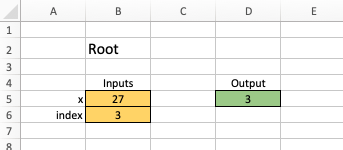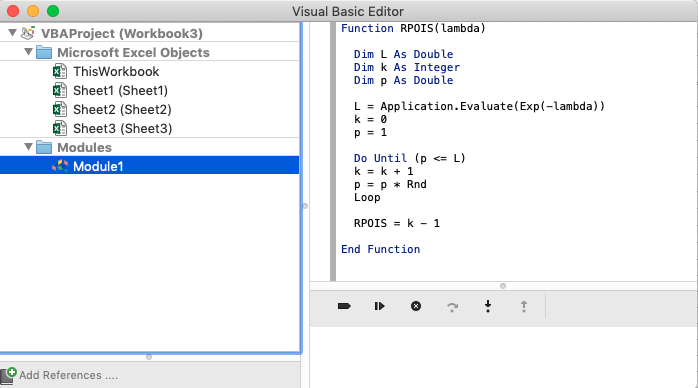## Building Your Own Functions in Excel

While using only Excel's available formulas in a worksheet won't let you simulate every function you can build with R, it can do more than one might think.

Consider the solve.quadratic function in R:

solve.quadratic = function(a,b,c) {
discriminant = sqrt(b^2-4*a*c)
solution1 = (-b-discriminant)/(2*a)
solution2 = (-b+discriminant)/(2*a)
return (c(solution1,solution2))
}

In Excel, instead of passing named arguments to the function like $a$, $b$, and $c$ and returning multiple outputs in a single vector, one can name specific cells to hold the arguments (i.e., inputs) for the function and do the same for the value or values to be returned (as outputs), as shown below:Granted, the arguments only have names $a$, $b$, and $c$ as far as the human reader of the worksheet is concerned. As one should probably already know by now, the "names" for these arguments are really their cell references (in the example above, that would be $B5$, $B6$, and $B7$).

We can use other cells (like $D5$ above) for intermediate calculations:

D5: "=B6^2-4*B5*B7"

before dealing with the final calculations that produce the output we seek:
F5: "=(-B6-D5)/(2*B5)"
F6: "=(-B6+D5)/(2*B5)"


#### Default Values for Arguments

There are even ways to integrate optional arguments into your calculations. The following worksheet, which calculates the $n^{th}$ root of $x$ for some user-supplied $x$ and $n$, provides an example. This worksheet uses a special conditional function $IF()$ about which there will be much, much more to say soon.

For now, however, simply note that the denominator of the second argument passed to $POWER()$ will be a 2 by default should the user leave the cell $B6$ blank -- but if the user puts a value in that cell, the denominator will be that user-entered value instead.D5: "=POWER(B5,1/IF(ISBLANK(B6),2,B6))"


#### What if the basic Excel functions aren't enough?

Sometimes there just isn't any way to do exactly what you want to do with only the basic functions available to you when you click the $f_x$ button in the formula bar at the top of the worksheet to bring up the "Formula Builder".

But... there is something lurking under the hood of Excel, that not everybody realizes. Excel has a built in programming language (i.e., Visual Basic) that you can use to do all of these things you can't otherwise do!

Programming in Visual Basic is a big topic -- one that is too big to develop here. However, just for fun, and so that you can see how to get to the place where one can "code" in Excel, open a blank workbook and hit Alt-F11 (on Windows) or opt-fn-F11 (on a Mac). This brings up the Visual Basic Editor. Right-click "VBA Project (...)" to insert a new module. Expanding the resulting folder and clicking on the module just added, the code editor window on the right becomes active:Just by typing the code shown in the right pane above, one can then immediately use this custom, user-defined function RPOIS(lambda) in your worksheet, which randomly generates a number of occurrences in accordance with a Poisson distribution where lambda is the expectation. (Compare this with the R code found at the bottom of this problem set.)

It looks like Excel has more power than we thought!